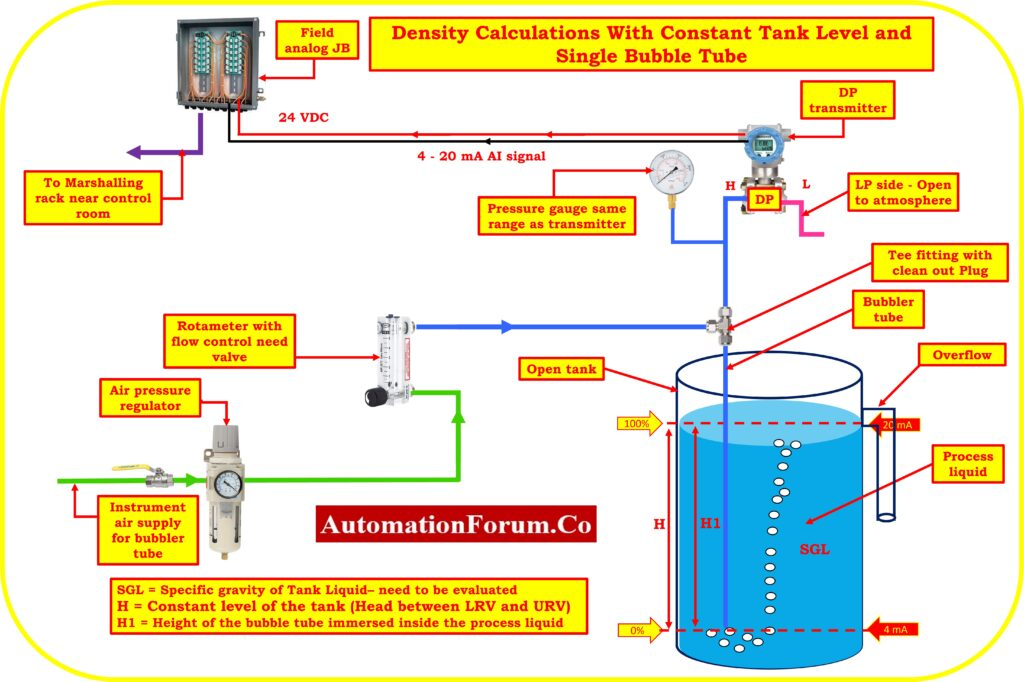# Bubbler method Liquid Density measurement Calculations with Constant Tank Level and Single Bubble TubeIn this section, examples of typical calculations for determining the liquid Density measurement Calculations with Constant Tank Level and single Bubble Tube Installation are given.

It is important to keep in mind that the upper-range values (URV) and lower-range values (LRV) relate to the highest and minimum measurements that are intended, respectively. The Calibrated Span is equal to the URV minus the LRV. The output at LRV greater than 0% represents the value of the suppression.

## Abbreviations

The following acronyms are utilized in this directive.

LRV = Lower-range value (a measurement that’s output is 4 mA).

URV = Upper-range value (a measurement with a 20 mA output).

SG = A liquid’s specific gravity (relative density). Water has a specific gravity of 1.00 in both traditional and SI systems.

HW= Equivalent Headlevel pressure of Water

## Formulas for Converting Specific Gravity for All Liquids

Specific gravity = Density [lb/ft3 ] / 62.4

Specific gravity = Density [kg/m3] / 1.00

H1 = Height of the bubble tube immersed inside the process liquid

SGL = Specific gravity of the liquid – need to be evaluated

SG1 = Minimum Specific gravity of the liquid

SG2 = Maximum Specific gravity of the liquid

H = Constant level of the tank (Head between LRV and URV)

Calibrated Range of the transmitter = LRV to URV

Where:

HW = Equivalent Head level pressure of Water

Span value of the transmitter = (H)*(SG2 – SG1)

Equivalent Head level pressure of Water (HW) at Lower range value

HW at LRV =(H*SG1) = Suppression

Equivalent Head level pressure of Water (HW) at Upper range value

HW at URV = (H*SG2)

## Example calculation -Density Calculations with Constant Tank Level and single Bubble Tube

The DP transmitter is mounted in an open tank with single bubble tube and also has the following specifications to determine density of the process liquid.

Process liquid Specific gravity varies between 1.0 and 1.8

Constant level of the tank is

H= 60 inches (Head between LRV and URV)

Find the calibration range of the transmitter for measuring the density?

So, SG1 = 1.0

SG2= 1.8

## Span value calculation

Span = (H)*(SG2 – SG1)

Span = (60)*(1.8 – 1.0)

Span = (60)*(0.8)

Span value of the transmitter = 48 inchH2O

## LRV calculation (4mA)

HW at LRV = (H*SG1)

HW at LRV = (60 *1.0)

HW at LRV= 60 inchH2O = Suppression

## URV calculation (20mA)

HW at URV = (H*SG2)

Hw at URV = 60 * (1.8)

HW at URV = 108 inchH2O

Calibration range of the transmitter = 60 to 108 inch of H2O

Scroll to Top